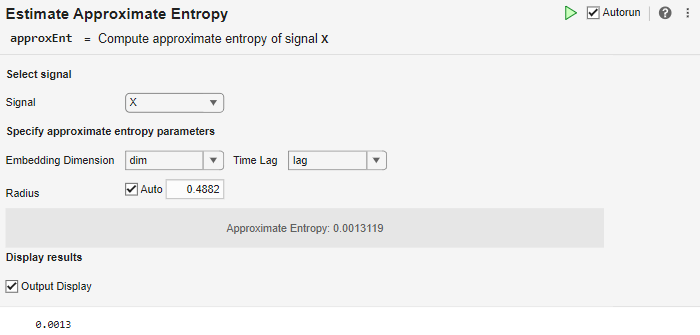Documentation

# Estimate Approximate Entropy

Interactively estimate the approximate entropy of a uniformly sampled signal in the Live Editor

## Description

Approximate entropy is a regularity statistic that quantifies the unpredictability of fluctuations in a time series. A relatively higher value of approximate entropy reflects the likelihood that similar patterns of observations are not followed by additional similar observations.To add the Estimate Approximate Entropy task to a live script in the MATLAB Editor:

• On the Live Editor tab, select Task > Estimate Approximate Entropy.

• In a code block in your script, type a relevant keyword, such as `approximate` or `approximate entropy`. Select `Estimate Approximate Entropy` from the suggested command completions.

## Examples

expand all

Use the Estimate Approximate Entropy task in the Live Editor to interactively estimate the approximate entropy of a uniformly sampled signal. Experiment with different values for lag, embedding dimension and radius. The task automatically generates code reflecting your selections. Open this example to see a preconfigured script containing the Estimate Approximate Entropy task.

For this example, consider '`approxEntData.mat`' which contains uniformly sampled signal `X`, embedding dimension `dim`, and the time delay `lag`.

`load('approxEntData.mat','X','dim','lag')`

To approximate entropy of the signal `X`, open the Estimate Approximate Entropy task in the Live Editor. On the Live Editor tab, select Task > Estimate Approximate Entropy. In the task, select signal `X`.Select `dim` for the Embedding Dimension and `lag` for the Time Lag dropdown menu respectively. If you do not know the embedding dimension and the time lag for your signal, use the Reconstruct Phase Space task to compute the values.Evaluate whether the value of approximate entropy is affected drastically by changing the Radius field and observe the change in value in the Live Editor output. You can toggle displaying the output of the approximate entropy value in the Live Editor output using the Output Display option.The task generates code in your live script. The generated code reflects the parameters and options you specify. To see the generated code, clickat the bottom of the task parameter area. The task expands to display the generated code.By default, the generated code uses `approxEnt` as the name of the output variable. To specify a different output variable name, enter a new name in the summary line at the top of the task. For instance, change the name to `aEntropy`.The task updates the generated code to reflect the new variable name, and the new variable `aEntropy` appears in the MATLAB workspace.

## Parameters

#### Select Signal

Select a uniformly sampled time-domain signal in array or timetable format. If the signal has multiple columns, the Estimate Approximate Entropy task computes the approximate entropy by treating it as a multivariate signal. If the signal is a row vector, then the Estimate Approximate Entropy task treats it as a univariate signal.

#### Specify Approximate Entropy Parameters

Specify the number of dimensions of phase space vectors as a scalar or vector from the MATLAB workspace. When you specify the embedding dimension as a scalar, then every column of the uniformly sampled signal is computed using the same embedding dimension value.

If you do not know the value of embedding dimension for your signal, then you can compute it using the Reconstruct Phase Space task.

Specify time lag between successive phase vectors as a scalar or vector from the MATLAB workspace. When you specify the time lag as a scalar, then the Estimate Approximate Entropy task uses the same time delay value to estimate the value of approximate entropy for all the columns of the uniformly sampled signal. If you specify the embedding dimension as a vector, then specify the time lag also as a vector of the same length.

If you do not know the value of time lag for your signal, then you can compute it using the Reconstruct Phase Space task.

Specify similarity criterion as a scalar. The similarity criterion, also called radius of similarity, is a tuning parameter that is used to identify a meaningful range in which fluctuations in data are to be considered similar.

#### Visualize Results

Toggle to display the value of approximate entropy in the Live Editor output.